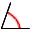The Rocscience International Conference 2021 Proceedings are now available. Read Now

# Measure Angle

The Measure Angleoption allows you to calculate the angle between any two points (planes) on the stereonet.

To use Measure Angle option:

1. Select Measure Anglefrom the Tools menu.
2. Click the LEFT mouse button at a point (pole) on the stereonet. Remember that the cursor coordinates are always displayed in the Status Bar.
3. Click the LEFT mouse button at a second point (pole).
4. A dialog will immediately appear, displaying the acute and obtuse angles between the two points (poles) selected.
5. In the Measure Angle dialog, the coordinates of the two selected points are also displayed, in the format corresponding to the current Convention (i.e., Trend / Plunge or Plane Vector format). If these graphically entered coordinates are not the exact coordinates intended, then simply enter the correct coordinates, and the angle between the new points will immediately be displayed.
6. The Measure Angle dialog can now simply be used as a calculator, by entering any pairs of coordinates, representing a pair of planes, or a pair of linear features. Each time a number is entered through the keyboard, or changed using the "up" or "down" arrows beside each coordinate entry field, the result is immediately calculated and displayed in the dialog.
7. When you are finished, select Close to exit from the Measure Angle option.

## Right Click Options

The Pole Snap option is available in the right-click menu, in steps 2 and 3 above, if you are using Measure Angle on a Pole Plot.

• Pole Snap – this will "snap" the cone to the pole nearest to the cursor location. Only applicable in Pole Vector Mode.

## Pitch Grid

The Pitch Grid option can also be used as a graphical method of estimating angles between features on the stereonet. However for precise answers, the Measure Angle option is recommended.

## Measuring Angle Between a Line and Plane

There is no option for directly measuring the angle between a line and a plane in Dips. However, you can do this using various workarounds. This example will consider a lineation (line) of 110/50 and a foliation (plane) of 175/50.

1. Use the Add Plane option to add a plane to the stereonet with dip/dip direction = 50/175.
2. Switch the coordinate convention to trend/plunge.
3. Use the Add Plane option to add the lineation with trend/plunge = 110/50.
4. Add a Pitch Grid plane which passes through the foliation pole, and the lineation point.
5. Use Measure Angle to measure the angle between the lineation point and the intersection of the foliation plane (great circle) and the pitch grid plane.
6. The angle should be approximately 17 degrees.